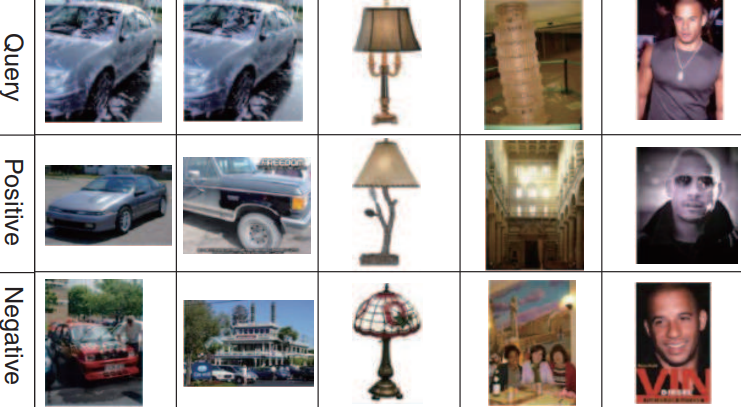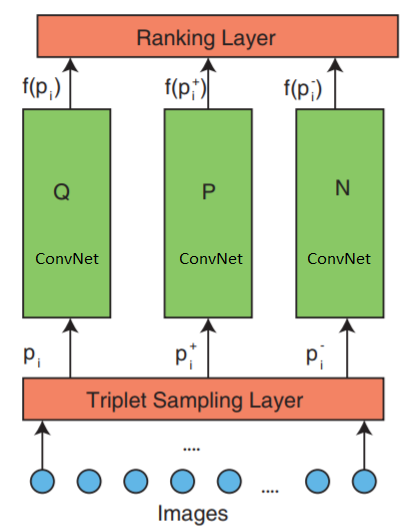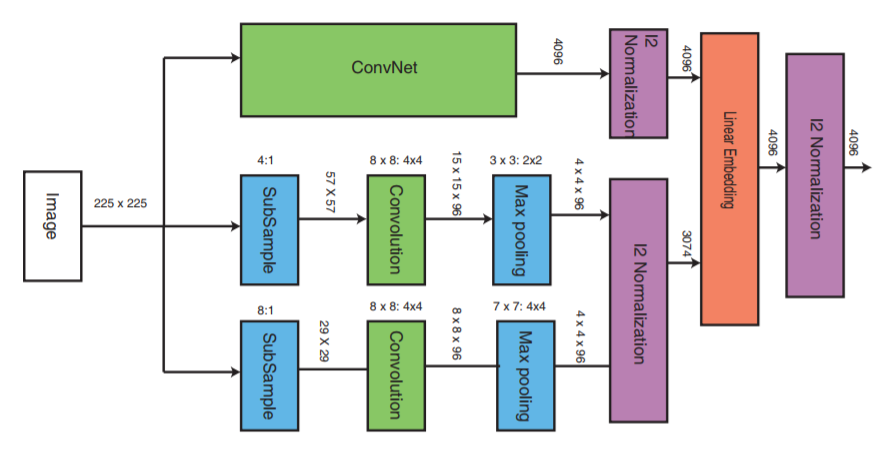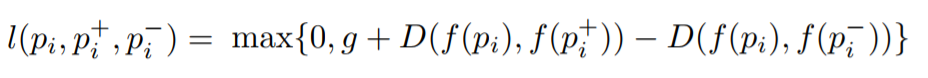# Deep ranking for comparing two images

Hello, Habr! I present to you the translation of the article “Image Similarity using Deep Ranking” by Akarsh Zingade.

## Deep Ranking Algorithm

The concept of " similarity of two images " was not introduced, so let's introduce this concept at least within the framework of the article.

The similarity of the two images is the result of comparing two images according to certain criteria. Its quantitative measure determines the degree of similarity between the intensity diagrams of two images. Using a similarity measure, some features describing the images are compared. As a measure of similarity, the following are usually used: Hamming distance, Euclidean distance, Manhattan distance, etc.

Deep Ranking - studies fine-grained similarity of images, characterizing the finely divided image similarity ratio using a set of triplets.

### What is a triplet?

The triplet contains the request image, the positive and negative image. Where a positive image is more like a request image than a negative.

An example of a set of triplets:The first, second, and third lines correspond to the image of the request. The second line (positive images) is more like request images than the third (negative images).

### Deep Ranking Network Architecture

The network consists of 3 parts: triplet sampling, ConvNet and a ranking layer.
The network accepts triplets of images as input. One image triplet contains a request image$inline p_i inline$positive image $inline p_i ^ + inline$ and negative image $inline p_i ^ - inline$that are independently transmitted to three identical deep neural networks.

The topmost ranking layer - evaluates the triplet loss function. This error is corrected in the lower layers in order to minimize the loss function.Let's now take a closer look at the middle layer:ConvNet can be any deep neural network (this article will discuss one of the implementation of the convolutional neural network VGG16). ConvNet contains convolutional layers, a maximum pool layer, local normalization layers, and fully connected layers.
The other two parts receive images with a reduced sampling rate and carry out the convolution stage and max pooling. Next, the normalization stage of the three parts takes place and at the end they are combined with a linear layer with subsequent normalization.

### Triplet Formation

There are several ways to create a triplet file, for example, use an expert assessment. But this article will use the following algorithm:

1. Each image in the class forms a request image.
2. Each image, except for the request image, will form a positive image. But you can limit the number of positive images for each image request
3. A negative image is randomly selected from any class that is not a request image class

### Triplet loss function

The goal is to train a function that assigns a small distance for the most similar images and a large one for different ones. This can be expressed as:Where l is the loss coefficient for triplets, g is the gap coefficient between the distance between two pairs of images: ($inline p_i inline$, $inline p_i ^ + inline$) and ($inline p_i inline$, $inline p_i ^ - inline$), f - embedding function that displays the image in a vector,$inline p_i inline$ Is the image of the request, $inline p_i ^ + inline$ Is a positive image, $inline p_i ^ - inline$Is a negative image, and D is the Euclidean distance between two Euclidean points.

### Deep Ranking Algorithm Implementation

Implementation with Keras.

Three parallel networks are used for query, positive and negative image.

There are three main parts to the implementation, these are:

1. Implementation of three parallel multiscale neural networks
2. Loss function implementation
3. Triplet generation

Learning three parallel deep networks will consume a lot of memory resources. Instead of three parallel deep networks that receive a request image, a positive and a negative image, these images will be fed sequentially to one deep neural network at the input of a neural network. The tensor transferred to the loss layer will contain an image attachment in each row. Each line corresponds to each input image in a packet. Since the request image, the positive image and the negative image are sequentially transmitted, the first line will correspond to the request image, the second to the positive image, and the third to the negative image, and then repeated until the end of the packet. Thus, the ranking layer receives an embedding of all images. After that, the loss function is calculated.

To implement the ranking layer, we need to write our own loss function, which will calculate the Euclidean distance between the request image and the positive image, as well as the Euclidean distance between the request image and the negative image.

Implementation of the loss calculation function
``````_EPSILON = K.epsilon()
def _loss_tensor(y_true, y_pred):
y_pred = K.clip(y_pred, _EPSILON, 1.0-_EPSILON)
loss =  tf.convert_to_tensor(0,dtype=tf.float32) # initialise the loss variable to zero
g = tf.constant(1.0, shape=, dtype=tf.float32) # set the value for constant 'g'
for i in range(0,batch_size,3):
try:
q_embedding = y_pred[i+0] # procure the embedding for query image
p_embedding =  y_pred[i+1] # procure the embedding for positive image
n_embedding = y_pred[i+2] # procure the embedding for negative image
D_q_p =  K.sqrt(K.sum((q_embedding - p_embedding)**2)) # calculate the euclidean distance between query image and positive image
D_q_n = K.sqrt(K.sum((q_embedding - n_embedding)**2)) # calculate the euclidean distance between query image and negative image
loss = (loss + g + D_q_p - D_q_n ) # accumulate the loss for each triplet
except:
continue
loss = loss/(batch_size/3) # Average out the loss
zero = tf.constant(0.0, shape=, dtype=tf.float32)
return tf.maximum(loss,zero)``````

The packet size should always be a multiple of 3. Since a triplet contains 3 images, and triplet images are transmitted sequentially (we send each image to a deep neural network sequentially)

The rest of the code is here

List of references
 Object Recognition from Local Scale-Invariant Features- www.cs.ubc.ca/~lowe/papers/iccv99.pdf

 Histograms of Oriented Gradients for Human Detection- courses.engr.illinois.edu/ece420/fa2017 /hog_for_human_detection.pdf

 Learning Fine-grained Image Similarity with Deep Ranking- static.googleusercontent.com/media/research.google.com/en//pubs/archive/42945.pdf

 ImageNet Classification with Deep Convolutional Neural Networks- papers.nips.cc/paper/4824-imagenet-classification-with-deep-convolutional-neural-networks.pdf

 Dropout: A Simple Way to Prevent Neural Networks from Overfitting- www.cs.toronto.edu/ ~ hinton / absps / JMLRdropout.pdf

 ImageNet: A Large-Scale Hierarchical Image Database-www.image-net.org/papers/imagenet_cvpr09.pdf

 Fast Multiresolution Image Querying- grail.cs.washington.edu/projects/query/mrquery.pdf

 Large-Scale Image Retrieval with Compressed Fisher Vectors- citeseerx .ist.psu.edu / viewdoc / download? doi = 10.1.1.401.9140 & rep = rep1 & type = pdf

 Beyond Bags of Features: Spatial Pyramid Matching for Recognizing Natural Scene Categories- ieeexplore.ieee.org/document/1641019

[10 ] Improved Consistent Sampling, Weighted Minhash and L1 Sketching- static.googleusercontent.com/media/research.google.com/en//pubs/archive/36928.pdf

 Large scale online learning of image similarity through ranking- jmlr. csail.mit.edu/papers/volume11/chechik10a/chechik10a.pdf

 Learning Fine-grained Image Similarity with Deep Ranking- users.eecs.northwestern.edu/~jwa368/pdfs/deep_ranking.pdf

 Image Similarity using Deep Ranking- medium.com/@akarshzingade/image-similarity-using -deep-ranking-c1bd83855978